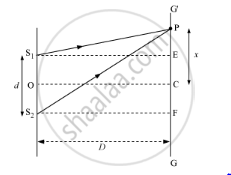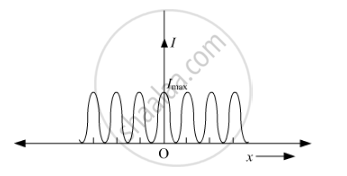# In Young'S Double-slit Experiment, Deduce the Condition for (A) Constructive and (B) Destructive Interferences at a Point on the Screen. Draw a Graph Showing Variation of Intensity in the Interference Pattern Against Position 'X' on the Screen. - Physics

(i) In Young's double-slit experiment, deduce the condition for (a) constructive and (b) destructive interferences at a point on the screen. Draw a graph showing variation of intensity in the interference pattern against position 'x' on the screen.

(b) Compare the interference pattern observed in Young's double-slit experiment with single-slit diffraction pattern, pointing out three distinguishing features.

#### Solution

Expression for Fringe Width in Young’s Double-Slit ExperimentLet S1 and S2 be two slits separated by a distance d. GG' is the screen at a distance D from the slits S1and S2. Point C is equidistant from both the slits. The intensity of light will be maximum at this point because the path difference of the waves reaching this point will be zero.

At point P, the path difference between the rays coming from the slits is given by

S1= S2P  − S1P

Now, S1 S2 = d, EF = d, and S2F = D

In ΔS2PF,

S_2P=[S_2F^2+PF^2]^(1/2)

S_2P=[D2+(x+d/2)^2]^(1/2)

=D[1+(x+d/2)^2/D^2]^(1/2)

Similarly, in ΔS1PE

S_1P=D[1+(x-d/2)^2/D^2]^(1/2)

:.S_2P-S_1P=D[1+1/2(x+d/2)^2/D^2)]-D[1+1/2(x-d/2)^2/D^2]

On expanding it binomially, we get

S_2P-S_1P=1/(2D)[4xd/2]=(xd)/D

For constructive interference, the path difference is an integral multiple of wavelengths, that is, path difference is .

:.nlambda=(xd)/D

x=(nlambdaD)/d

where n = 0, 1, 2, 3, 4, …

Similarly, for destructive interference,

x_n=(2n-1)lambda/2D/d

Graph of Intensity Distribution in Young’s Double-Slit Experiment(ii) On comparing the interference pattern observed in Young's double slit experiment (interference) with single-slit diffraction pattern (diffraction), we can have three distinguishing features:

In the interference pattern, all the bright fringes have the same intensity. In a diffraction pattern, all the bright fringes are not of the same intensity

In the interference pattern, the dark fringe has zero or very small intensity so that the bright and dark fringes can easily be distinguished. In diffraction pattern, all the dark fringes are not of zero intensity

In the interference pattern, the widths of all the fringes are almost the same, whereas in diffraction pattern, the fringes are of different widths

Concept: Interference of Light Waves and Young’S Experiment
Is there an error in this question or solution?